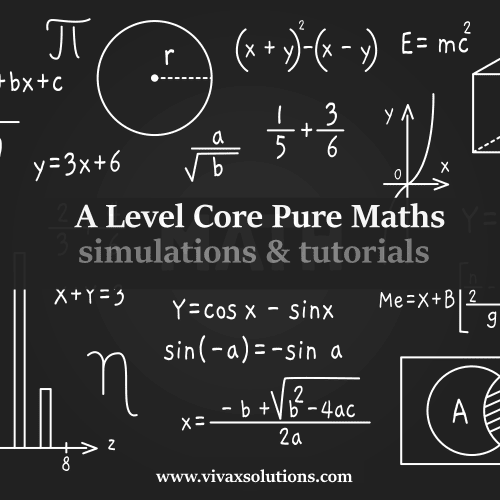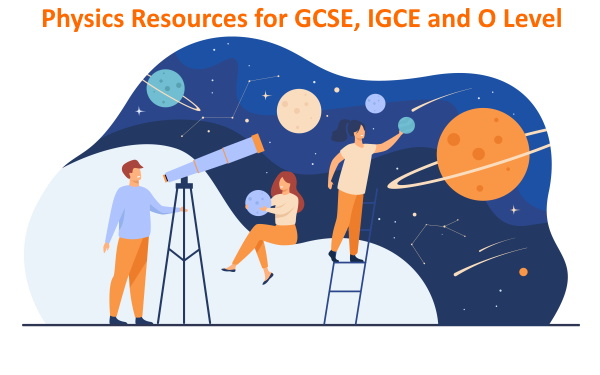## Posts

Showing posts from October 30, 2022

### A Level further maths: equation of a half line - complex numbersThe following simulation, which is fully interactive, shows you how to  derive the equation of a half line . A half line , by definition,  is a line that stretches from a point in one direction up to infinity.  You may change the position of the arbitrary point, denoted by Z, and the equation of the half-line will be automatically derived.  🎕 I am immensely thankful to the GeoGebra foundation for providing the programmers with this platform to play with amazing tools to  learn, share and above all, have fun - at the same time.

### Locus of a point: A Level further maths - complex numbersWith the following simulation, you can find out the equation of the locus of a point that moves in such a way that Argument(Z - z1) = Θ. The locus of the point is a half line - a line that starts from a point and moves only in one direction from it up to infinity.  You can change the position of Z - along with the argument - and find the equation of the half -line.

### Resultant Moment of Forces - GCSE, IGCSE and A Level physicsWith the following simulation, you can practise the moment of forces interactively. Moment of a force = force X perpendicular distance from the pivot The resultant moment or the total moment is the algebraic sum of the moments  of forces.  In the simulation, the clockwise moment is considered as positive. To increase the magnitude of a force, drag the end of each force/s up or down. The force that is closest to A can even be moved diagonally. Move the forces and study the way the total or resultant moment changes.

### A Level Core Maths: complex numbers and lociThe above simulation can be used to learn the locus of a point, under given conditions. Just move the point, Z, using the mouse and watch the appearance and disappearance of the shaded region. Alternatively, you can use the play button at the bottom-right corner too.